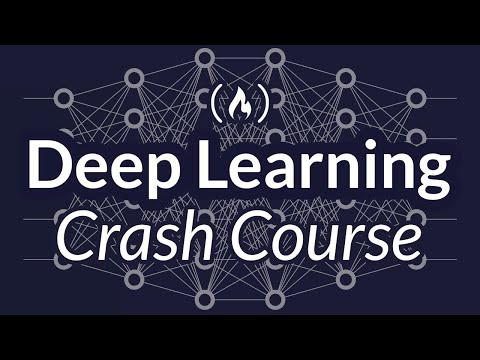Learn the fundamental concepts and terminology of Deep Learning, a sub-branch of Machine Learning. This course is designed for absolute beginners with no experience in programming. You will learn the key ideas behind deep learning without any code.

You’ll learn about Neural Networks, Machine Learning constructs like Supervised, Unsupervised and Reinforcement Learning, the various types of Neural Network architectures, and more.

✏️ Course developed by Jason Dsouza. Check out his YouTube channel: https://www.youtube.com/channel/UCeD0yJbElGy09DLucx60Xrg

⭐️ Course Contents ⭐️
⌨️ (0:00) Introduction
⌨️ (1:18) What is Deep Learning
⌨️ (5:25) Introduction to Neural Networks
⌨️ (6:12) How do Neural Networks LEARN?
⌨️ (12:06) Core terminologies used in Deep Learning
⌨️ (12:11) Activation Functions
⌨️ (22:36) Loss Functions
⌨️ (23:42) Optimizers
⌨️ (30:10) Parameters vs Hyperparameters
⌨️ (32:03) Epochs, Batches & Iterations
⌨️ (34:24) Conclusion to Terminologies
⌨️ (35:18) Introduction to Learning
⌨️ (35:34) Supervised Learning
⌨️ (40:21) Unsupervised Learning
⌨️ (43:38) Reinforcement Learning
⌨️ (46:25) Regularization
⌨️ (51:25) Introduction to Neural Network Architectures
⌨️ (51:37) Fully-Connected Feedforward Neural Nets
⌨️ (54:05) Recurrent Neural Nets
⌨️ (1:04:40) Convolutional Neural Nets
⌨️ (1:08:07) Introduction to the 5 Steps to EVERY Deep Learning Model
⌨️ (1:08:23) 1. Gathering Data
⌨️ (1:11:27) 2. Preprocessing the Data
⌨️ (1:19:05) 3. Training your Model
⌨️ (1:19:33) 4. Evaluating your Model
⌨️ (1:19:55) 5. Optimizing your Model’s Accuracy
⌨️ (1:25:15) Conclusion to the Course